Home » Comparison Instructions in PLC Programming
PLC Tutorials

# Comparison Instructions in PLC Programming

Comparison instructions in PLC are used to test pairs of values to condition the logical continuity of a rung.

Thus, comparison instructions would seldom, if ever, be the last instruction on a rung.

## Types of Comparison Instructions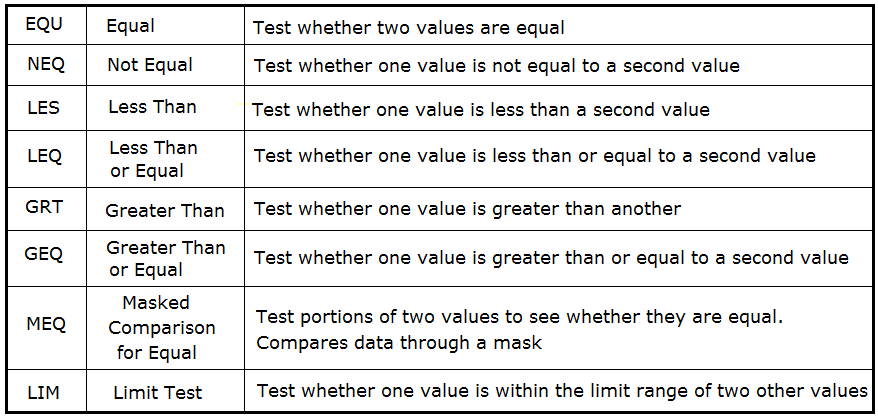As an example, suppose a LES instruction is presented with two values. If the first value is less than the second, then the comparison instruction is true.

## Equal (EQU) Instruction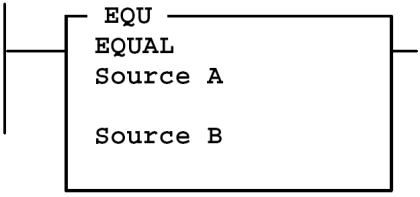Use the EQU instruction to test whether two values are equal. If source A and source B are equal, the instruction is logically true. If these values are not equal, the instruction is logically false.

Source A must be an address.

Source B can be either a program constant or an address.

Values are stored in two’s complementary form.

## Not Equal (NEQ) Instruction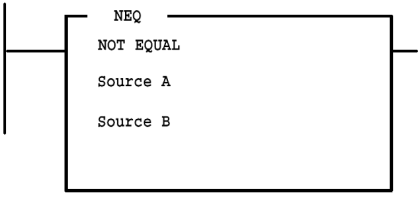Use the NEQ instruction to test whether two values are not equal.

If source A and source B are not equal, the instruction is logically true.

Source A must be an address.

Source B can be either a program constant or an address.

Values are stored in two’s complementary form.

## Less Than (LES) InstructionUse the LES instruction to test whether one value (source A) is less than another (source B).

If source A is less than the value at source B, the instruction is logically true.

Source A must be an address.

Source B can be either a program constant or an address.

Values are stored in two’s complementary form.

## Less Than or Equal (LEQ) Instruction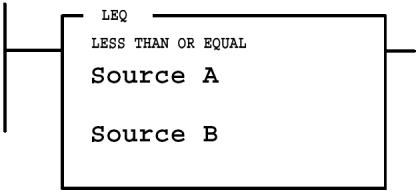Use the LEQ instruction to test whether one value (source A) is less than or equal to another (source B).

If the value at source A is less than or equal to the value at source B, the instruction is logically true.

Source A must be an address.

Source B can be either a program constant or an address.

Values are stored in two’s complementary form.

## Greater Than (GRT) Instruction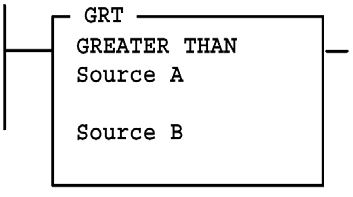Use the GRT instruction to test whether one value (source A) is greater than another (source B).

If the value at source A is greater than the value at source B, the instruction is logically true.

## Greater Than Or Equal (GEQ) Instruction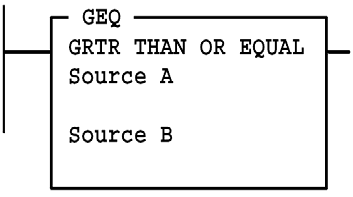Use the GEQ instruction to test whether one value (source A) is greater than or equal to another (source B).

If the value at source A is greater than or equal to the value at source B, the instruction is logically true.

## Masked Comparison for Equal (MEQ)Use the MEQ instruction to compare data at a source address with data at a compare address.

The Use of this instruction allows portions of the data to be masked by a separate word.

Source is the address of the value you want to compare.

Compare is an integer value or the address of the reference.

If the 16 bits of data at the source address are equal to the 16 bits of data at the compare address (less masked bits), the instruction is true.

The instruction becomes false as soon as it detects a mismatch.

## Limit Test (LIM) InstructionUse the LIM instruction to test for values within or outside a specified range, depending on how you set the limits.

The Low Limit, Test, and High Limit values can be word addresses or constants, restricted to the following combinations:

• If the Test parameter is a program constant, both the Low Limit and High Limit parameters must be word addresses.
• If the Test parameter is a word address, the Low Limit and High Limit parameters can be either a program constant or a word address.

### True/False Status of the LIM Instruction

If the Low Limit has a value equal to or less than the High Limit, the instruction is true when the Test value is between the limits or is equal to either limit.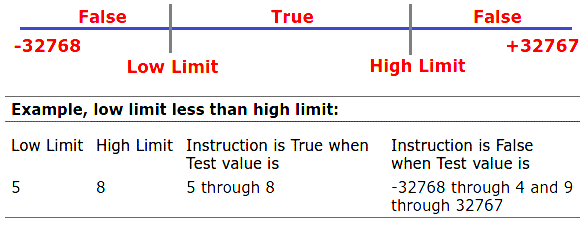If the Low Limit has a value greater than the High Limit, the instruction is false when the Test value is between the limits.#### Articles You May Like :

JUMP Instruction in PLC Programs

Master Control Reset (MCR) in PLC

One Shot Rising & Falling Instructions

Sequence and Logic Control in PLC

Scaling with Parameters Instruction

#### PLC Motor Control Ladder Logic ProgrammingSeptember 4, 2019 at 8:14 pm

Dear Sir
This is the fantastic Documents for Study & Learning you have given.
Indeed there are lots of lessons to learn.
Kindly, keep on teaching us so that we can upgrade the human civilization.
With RegardsSeptember 17, 2019 at 3:28 am

The logical instructional tools will help us greatly in developing our skills between PLC and SCADA …

keep providing these tools

graciously

This website uses cookies to improve your experience. We'll assume you're ok with this, but you can opt-out if you wish. Accept Read More

WordPress Image Lightbox

Send this to a friend